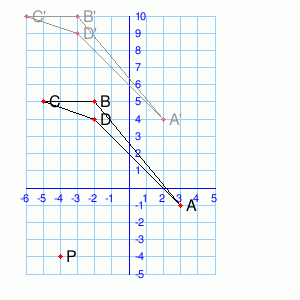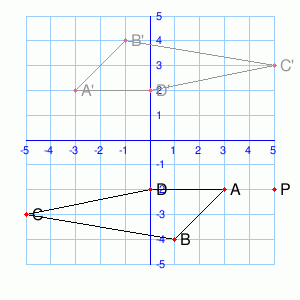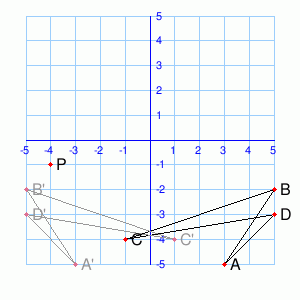# Math Practice Topic: Transformations 2

 Description: Translation, rotation, reflection. Adaptive Learning Progression: Problems become more complex. Start using MathScore for free

## Sample Levels (out of 6)

 1What type of transform takes ABCD to A'B'C'D'?        Translation        Rotation        Reflection 2What type of transform takes ABCD to A'B'C'D'?        Translation        Rotation        Reflection 3What type of transform takes ABCD to A'B'C'D'?        Translation        Rotation        Reflection

 1 Given a reflection across  x = 2 , followed by a reflection across  y = 2 , the combined transformation is a        translation        rotation If this is a translation, give the translation components; if it is a rotation, give the coordinates of the pivot point: (),( ) 2 Given a reflection across  y = -1 , followed by a reflection across  x = 1 , the combined transformation is a        translation        rotation If this is a translation, give the translation components; if it is a rotation, give the coordinates of the pivot point: (),( )